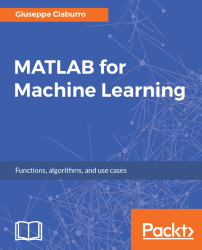•#### MATLAB for Machine Learning#### Overview of this book

MATLAB is the language of choice for many researchers and mathematics experts for machine learning. This book will help you build a foundation in machine learning using MATLAB for beginners. You’ll start by getting your system ready with t he MATLAB environment for machine learning and you’ll see how to easily interact with the Matlab workspace. We’ll then move on to data cleansing, mining and analyzing various data types in machine learning and you’ll see how to display data values on a plot. Next, you’ll get to know about the different types of regression techniques and how to apply them to your data using the MATLAB functions. You’ll understand the basic concepts of neural networks and perform data fitting, pattern recognition, and clustering analysis. Finally, you’ll explore feature selection and extraction techniques for dimensionality reduction for performance improvement. At the end of the book, you will learn to put it all together into real-world cases covering major machine learning algorithms and be comfortable in performing machine learning with MATLAB.
Title PageCreditsForewordwww.PacktPub.comCustomer FeedbackPrefaceFree Chapter
Getting Started with MATLAB Machine LearningImporting and Organizing Data in MATLABFrom Data to Knowledge DiscoveryFinding Relationships between Variables - Regression TechniquesPattern Recognition through Classification AlgorithmsIdentifying Groups of Data Using Clustering MethodsSimulation of Human Thinking - Artificial Neural NetworksImproving the Performance of the Machine Learning Model - Dimensionality ReductionMachine Learning in Practice## Chapter 6. Identifying Groups of Data Using Clustering Methods

Clustering methods are designed to find hidden patterns or groupings in a dataset. Unlike the supervised learning methods covered in previous chapters, these algorithms identify a grouping without any label to learn from through the selection of clusters based on similarities between elements.

This is an unsupervised learning technique that groups statistical units to minimize the intragroup distance and maximize the intergroup distance. The distance between the groups is quantified by means of similarity/dissimilarity measures defined between the statistical units.

To perform cluster analysis, no prior interpretative model is required. In fact, unlike other multivariate statistical techniques, this one does not make an apriori assumption on the existing fundamental typologies that may characterize the observed sample. This, however, occurs in the case of discriminating analysis, which makes it possible to split a set of individuals...